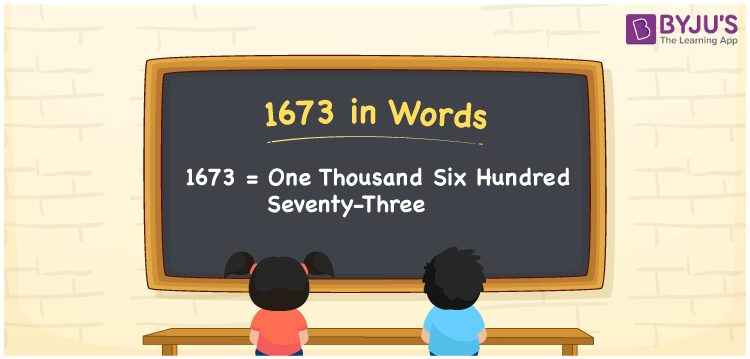# 1673 in words

1673 in words is written as One Thousand Six Hundred and Seventy Three. 1673 represents the count or value. The article on Counting Numbers can give you an idea about count or counting. The number 1673 is used in expressions that relate to money, distance, length, year and others. Let us consider an example for 1673. ”One Thousand Six Hundred and Seventy Three was not a leap year.”

 1673 in words One Thousand Six Hundred and Seventy Three One Thousand Six Hundred and Seventy Three in Numbers 1673

## 1673 in English Words## How to Write 1673 in Words?

We can convert 1673 to words using a place value chart. The number 1673 has 4 digits, so let’s make a chart that shows the place value up to 4 digits.

 Thousands Hundreds Tens Ones 1 6 7 3

Thus, we can write the expanded form as:

1 × Thousand + 6 × Hundred + 7 × Ten + 3 × One

= 1 × 1000 + 6 × 100 + 7 × 10 + 3 × 1

= 1673

= One Thousand Six Hundred and Seventy Three.

1673 is the natural number that is succeeded by 1672 and preceded by 1674.

1673 in words – One Thousand Six Hundred and Seventy Three.

Is 1673 an odd number? – Yes.

Is 1673 an even number? – No.

Is 1673 a perfect square number? – No.

Is 1673 a perfect cube number? – No.

Is 1673 a prime number? – No.

Is 1673 a composite number? – Yes.

## Solved Example

1. Write the number 1673 in expanded form

Solution: 1 × 1000 + 6 × 100 + 7 × 10 + 3 × 1

We can write 1673 = 1000 +600 +70 + 3

= 1 × 1000 + 6 × 100 + 7 × 10 + 3 × 1

## Frequently Asked Questions on 1673 in words

Q1

### How to write 1673 in words?

1673 in words is written as One Thousand Six Hundred and Seventy Three.
Q2

### Is 1673 a perfect square number?

No. 1673 is not a perfect square number.
Q3

### Is 1673 a prime number?

No. 1673 is not a prime number.